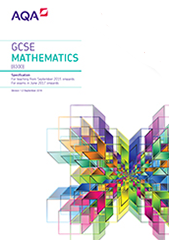# 3.5 Probability

## P1

Basic foundation content

Higher content only

record, describe and analyse the frequency of outcomes of probability experiments using tables and frequency trees

Notes: probabilities should be written as fractions, decimals or percentages.

## P2

Basic foundation content

Higher content only

apply ideas of randomness, fairness and equally likely events to calculate expected outcomes of multiple future experiments

## P3

Basic foundation content

Higher content only

relate relative expected frequencies to theoretical probability, using appropriate language and the 0 to 1 probability scale

## P4

Basic foundation content

Higher content only

apply the property that the probabilities of an exhaustive set of outcomes sum to 1

apply the property that the probabilities of an exhaustive set of mutually exclusive events sum to 1

## P5

Basic foundation content

Higher content only

understand that empirical unbiased samples tend towards theoretical probability distributions, with increasing sample size

## P6

Basic foundation content

Higher content only

enumerate sets and combinations of sets systematically, using tables, grids, Venn diagrams

including using tree diagrams

## P7

Basic foundation content

Higher content only

construct theoretical possibility spaces for single and combined experiments with equally likely outcomes and use these to calculate theoretical probabilities

## P8

Basic foundation content

Higher content only

calculate the probability of independent and dependent combined events, including using tree diagrams and other representations, and know the underlying assumptions

Notes: including knowing when to add and when to multiply two or more probabilities.

## P9

Basic foundation content